A Transportation Problem

You can easily translate the symbolic formulation of a problem into the OPTMODEL procedure. Consider the transportation problem, which is mathematically modeled as the following linear programming problem: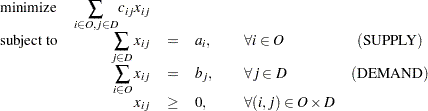where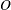is the set of origins,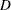is the set of destinations,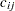is the cost to transport one unit fromto,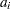is the supply of origin,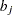is the demand of destination, and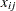is the decision variable for the amount of shipment fromto.

Here is a very simple example. The cities in the setof origins are Detroit and Pittsburgh. The cities in the setof destinations are Boston and New York. The cost matrix, supply, and demand are shown in Table 4.2.

 Boston New York Supply Detroit 30 20 200 Pittsburgh 40 10 100 Demand 150 150

The problem is compactly and clearly formulated and solved by using the OPTMODEL procedure with the following statements:

```proc optmodel;

/* specify parameters */
set O={'Detroit','Pittsburgh'};
set D={'Boston','New York'};
number c{O,D}=[30 20
40 10];
number a{O}=[200 100];
number b{D}=[150 150];
/* model description */
var x{O,D} >= 0;
min total_cost = sum{i in O, j in D}c[i,j]*x[i,j];
constraint supply{i in O}: sum{j in D}x[i,j]=a[i];
constraint demand{j in D}: sum{i in O}x[i,j]=b[j];
/* solve and output */
solve;
print x;
```

The output is shown in Figure 4.4.

Figure 4.4 Solution to the Transportation Problem
The OPTMODEL Procedure

Problem Summary
Objective Sense Minimization
Objective Function total_cost
Objective Type Linear

Number of Variables 4
Bounded Above 0
Bounded Below 4
Bounded Below and Above 0
Free 0
Fixed 0

Number of Constraints 4
Linear LE (<=) 0
Linear EQ (=) 4
Linear GE (>=) 0
Linear Range 0

Constraint Coefficients 8

Solution Summary
Solver Dual Simplex
Objective Function total_cost
Solution Status Optimal
Objective Value 6500
Iterations 0

Primal Infeasibility 0
Dual Infeasibility 0
Bound Infeasibility 0

x
Boston New York
Detroit 150 50
Pittsburgh 0 100